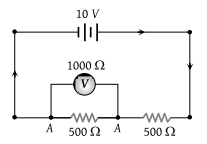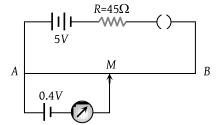The length of a wire of a potentiometer is 100 cm, and the emf of its standard cell is E volt. It is employed to measure the e.m.f of a battery whose internal resistance is 0.5 Ω. If the balance point is obtained at l = 30 cm from the positive end, the e.m.f. of the battery is :

(1) $\frac{30E}{100}$

(2) $\frac{30E}{100.5}$

(3) $\frac{30E}{\left(100-0.5\right)}$

(4) $\frac{30\left(E-0.5i\right)}{100}$, where i is the current in the potentiometer

Concept Questions :-

Grouping of cells
High Yielding Test Series + Question Bank - NEET 2020

Difficulty Level:

What is the reading of the voltmeter in the following figure?(1) 3 V

(2) 2 V

(3) 5 V

(4) 4 V

Concept Questions :-

Kirchoff's voltage law
High Yielding Test Series + Question Bank - NEET 2020

Difficulty Level:

The current flowing in a coil of resistance 90 Ω is to be reduced by 90%. What value of resistance should be connected in parallel with it

(1) 9 Ω

(2) 90 Ω

(3) 1000 Ω

(4) 10 Ω

High Yielding Test Series + Question Bank - NEET 2020

Difficulty Level:

A galvanometer of 50 ohm resistance has 25 divisions. A current of 4 × 10–4 ampere gives a deflection of one division. To convert this galvanometer into a voltmeter having a range of 25 volts, it should be connected with a resistance of :

(1) 2500 Ω as a shunt

(2) 2450 Ω as a shunt

(3) 2550 Ω in series

(4) 2450 Ω in series

Concept Questions :-

Meter bridge and potentiometer
High Yielding Test Series + Question Bank - NEET 2020

Difficulty Level:

In a metre bridge experiment, the null point is obtained at 20 cm from one end of the wire when resistance X is balanced against another resistance Y. If X < Y, then where will be the new position of the null point from the same end, if one decides to balance a resistance of 4X against Y

(1) 50 cm

(2) 80 cm

(3) 40 cm

(4) 70 cm

Concept Questions :-

Meter bridge and potentiometer
High Yielding Test Series + Question Bank - NEET 2020

Difficulty Level:

In given figure, the potentiometer wire AB has a resistance of 5 Ω and length 10 m. The balancing length AM for the emf of 0.4 V is :(1) 0.4 m

(2) 4 m

(3) 0.8 m

(4) 8 m

Concept Questions :-

Meter bridge and potentiometer
High Yielding Test Series + Question Bank - NEET 2020

Difficulty Level:

In a potentiometer experiment, the balancing with a cell is at length 240 cm. On shunting the cell with a resistance of 2 Ω, the balancing length becomes 120 cm. The internal resistance of the cell is :

1. 4 Ω

2. 2 Ω

3. 1 Ω

4. 0.5 Ω

Concept Questions :-

Meter bridge and potentiometer
High Yielding Test Series + Question Bank - NEET 2020

Difficulty Level:

If the resistance of voltmeter is 10000Ω and resistance of ammeter is 2Ω then find R when voltmeter reads 12V and ammeter reads 0.1 A :

(1) 118 Ω

(2) 120 Ω

(3) 124 Ω

(4) 114Ω

Concept Questions :-

Kirchoff's voltage law
High Yielding Test Series + Question Bank - NEET 2020

Difficulty Level:

Potentiometer wire of length 1 m is connected in series with 490 Ω resistance and 2V battery. If 0.2 mV/cm is the potential gradient, then the resistance of the potentiometer wire is :

(1) 4.9 Ω

(2) 7.9 Ω

(3) 5.9 Ω

(4) 6.9 Ω

Concept Questions :-

Meter bridge and potentiometer
High Yielding Test Series + Question Bank - NEET 2020

Difficulty Level:

In an electrical cable, there is a single wire of radius 9 mm of copper. Its resistance is 5 Ω. The cable is replaced by 6 different insulated copper wires, the radius of each wire is 3 mm. Now the total resistance of the cable will be :

(1) 7.5 Ω

(2) 45 Ω

(3) 90 Ω

(4) 270 Ω

Concept Questions :-

Combination of resistors